## Introduction

Consider the following: x2 + 2x = 20

Is this an algebraic equation? Yes. What is the difference between x2 + 2x = 20 and x + 4 = 15?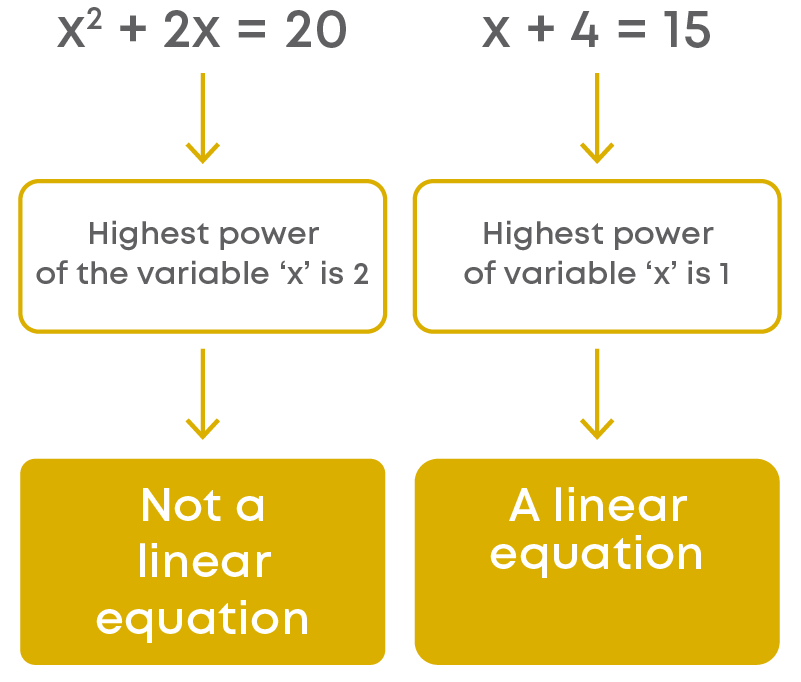A linear equation in one variable will be of the form ax + b = 0, where ‘a’ and ‘b’ are rational numbers and ‘x’ is a variable. Let us see some examples of linear equations in one variable:

• 2x + 13 = 13
• 2y + 6 = -4
• 3z = 36

Now, consider the following equation: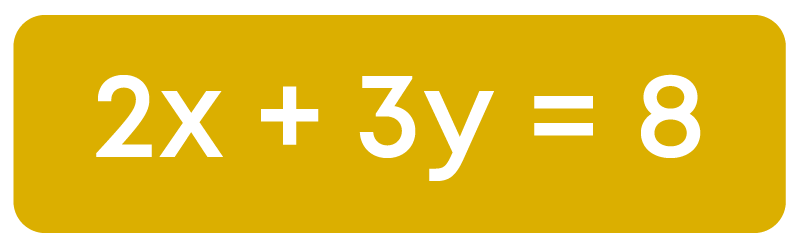Is this a linear equation? Yes! The equation above has two variables, ‘x’ and ‘y’. Such equations are called linear equations in two variables.

An equation of the form ax + by + c = 0, where a, b, and c are real numbers, such that ‘a’ and ‘b’ are both not equal to zero, is called a linear equation in two variables.

Where do we use linear equation in two variables? Let us watch the video below.

#### Concepts

The chapter ‘Linear Equations in Two Variables’ covers the following concepts:

#### Linear Equations

A linear equation is an algebraic statement with an equality sign in it.

• The expression on the left of the equal sign is called the LHS (left-hand side) and the expression on the right of the equal sign is called the RHS (right-hand side).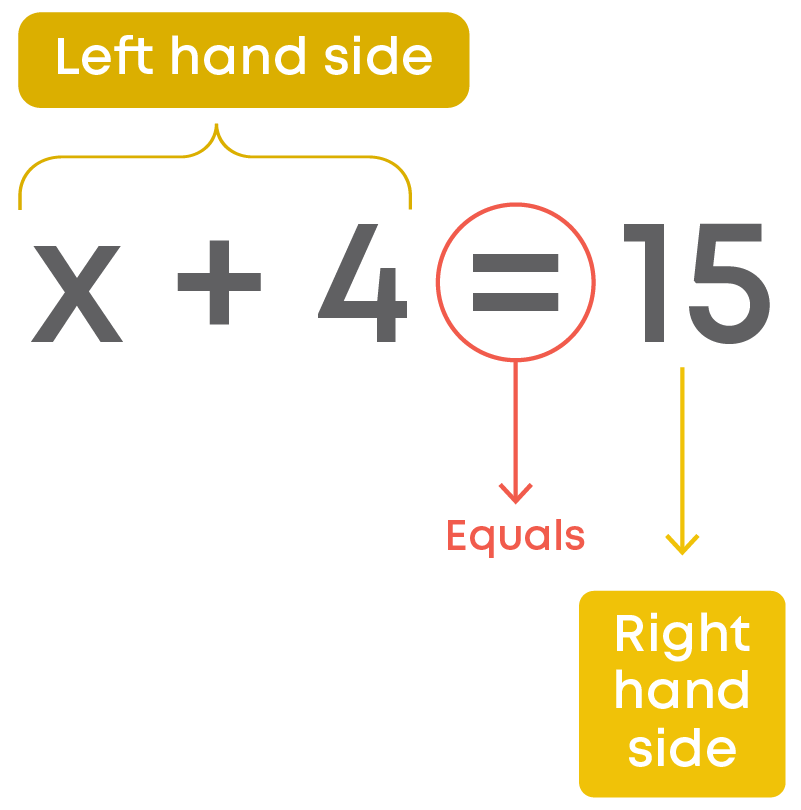• In a linear equation, the LHS is equal to RHS, for a certain value of the variable in the equation.
• This value will be the solution of the linear equation.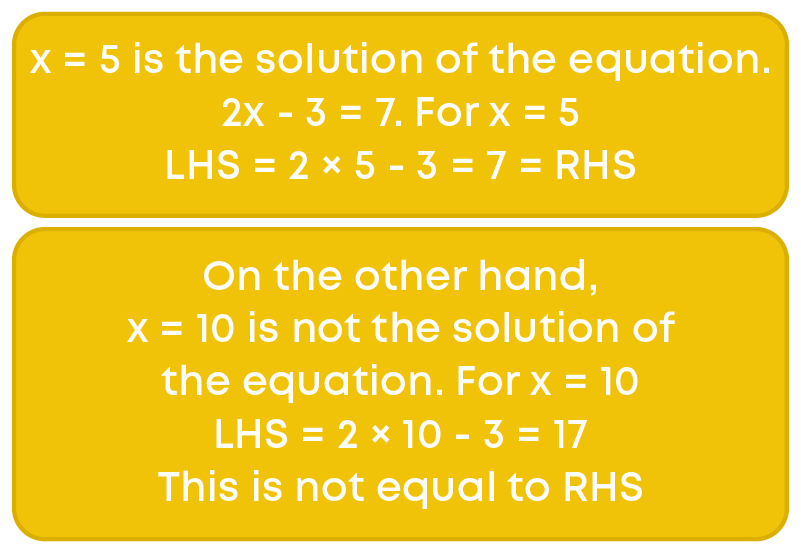Examples of linear equations in one variable: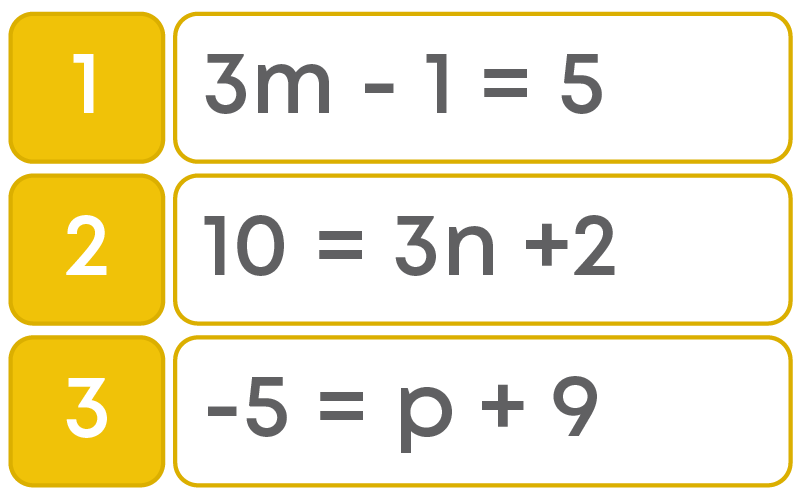Some examples of linear equations in two variables: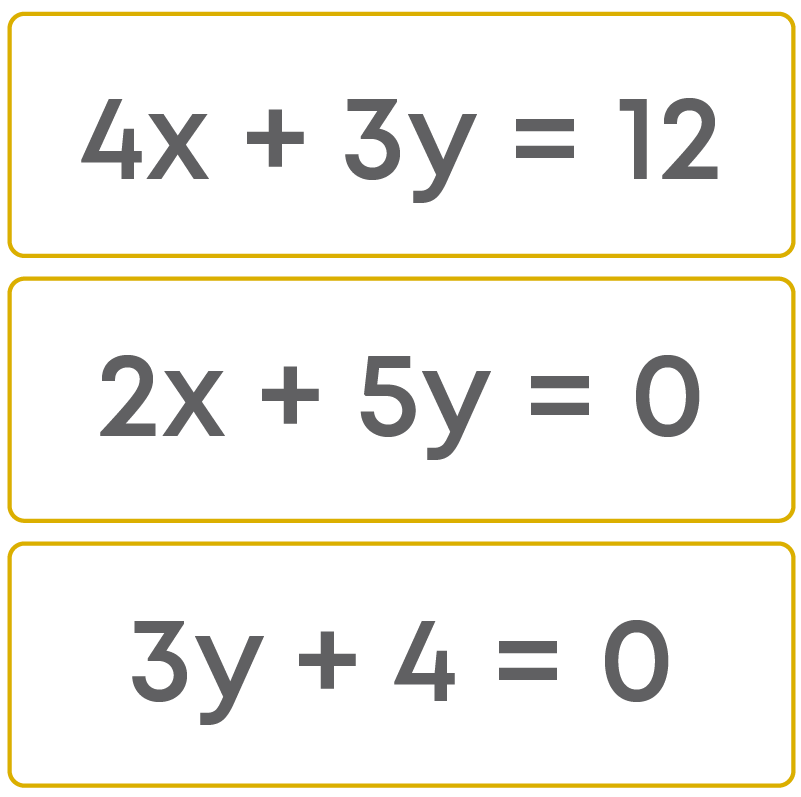Let us consider the situation given below.

Ritu and Rakesh went to a stationery shop and purchased notebooks, pens, crayons, and erasers. Then they together paid ₹275. How will you express this situation?

What is the amount of money spent by Ritu and Rakesh individually? In this situation, there are two unknowns.

The first one is the amount of money spent by Ritu and the second one is the amount of money spent by Rakesh.

Let us consider the amount of money spent by Ritu as x and the amount of money spent by Rakesh as y.

So, we can express this as x + y = 275. This is an example of a linear equation in two variables.

Let us learn how to write linear equations in the general/standard form, i.e., ax + by + c = 0:

Consider 3 = 2x + y

We can transpose / move 3 to the other side and rewrite the equation as:

2x + y – 3 = 0 or 2x + 1y – 3 = 0

Now, let us compare it with ax + by + c = 0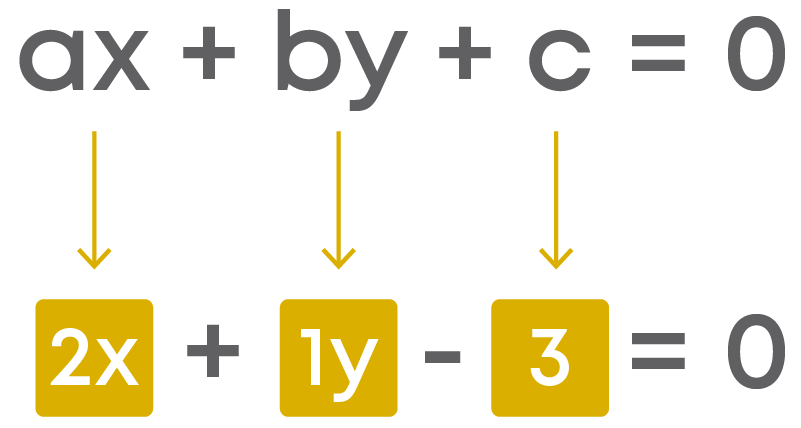Therefore, a = 2, b = 1, and c = -3

Now consider the given statement:

The cost of a notebook is twice the cost of a pen.

Let us see how to represent this mathematically. Let the cost of a notebook = ₹u. Now, the cost of the pen is also unknown. We need another variable to represent the cost.

Let the cost of a pen = ₹v

According to the question, the cost of a notebook is twice the cost of a pen, i.e.,

Cost of a notebook = 2 × Cost of a pen

u = 2 × v

u = 2v

u – 2v = 0 is the linear equation in two variables to represent the statement ‘the cost of a notebook is twice the cost of a pen.’

We follow the given strategy to represent real life statements in linear equation form: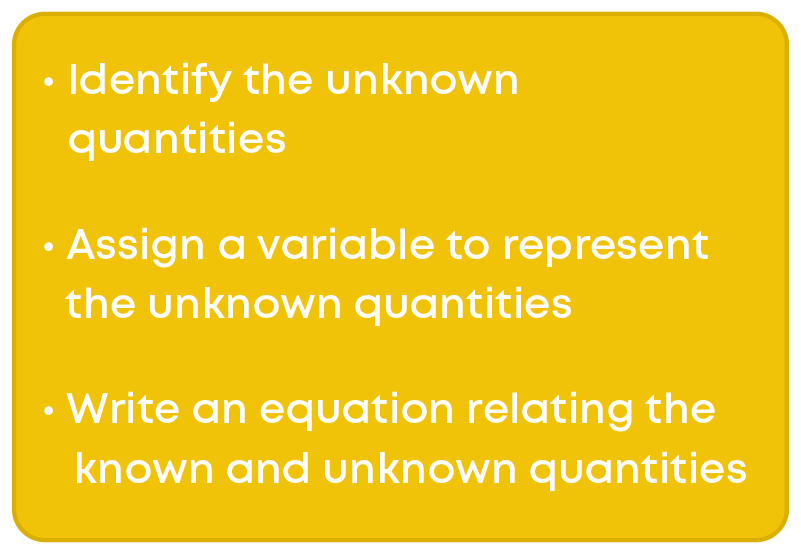Let us know the meaning of satisfying the equation or solution of an equation. For example, consider the linear equation.

x + 2 = 7

In the given equation, we know that left hand side of the equation is equal to the righthand side of the equation. So, think of a number which when substituted in place of ‘x’ gives the total value of LHS as 7.

Let us put 5 in place of ‘x,’ we get:

5 + 2 = 7

7 = 7 is true, so x = 5 is a solution of the given equation.

Observe some more examples: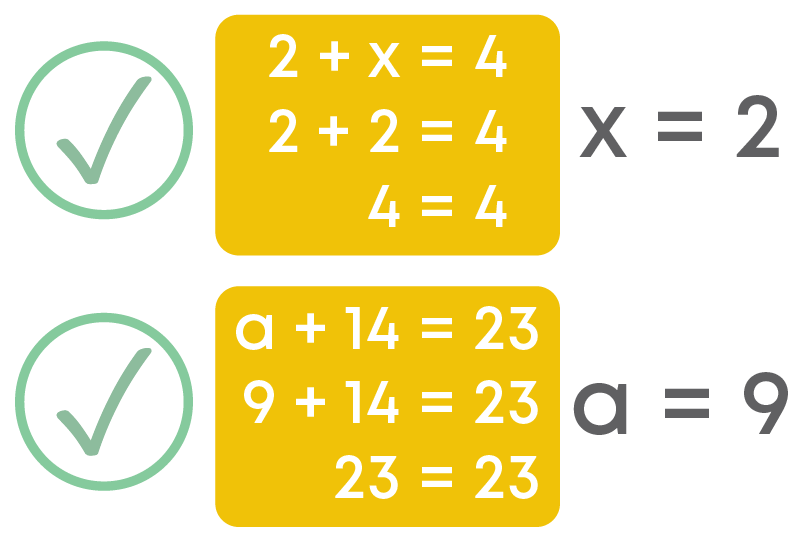So, 2 is a solution of the equation 2 + x = 4 and 9 is a solution of the equation a + 14 = 23.

In simple words we can say that solution of an equation is a value of the variable that makes the equation true.

Observe the conversation between two school friends, Rahul, and Abhay.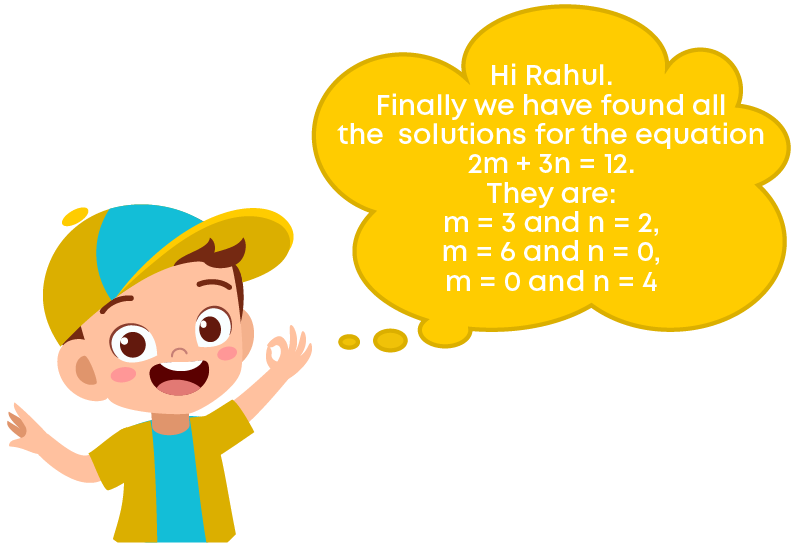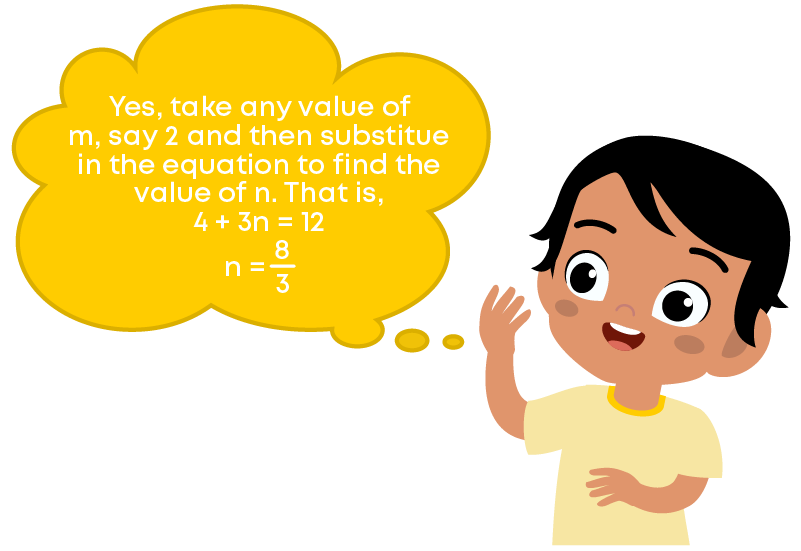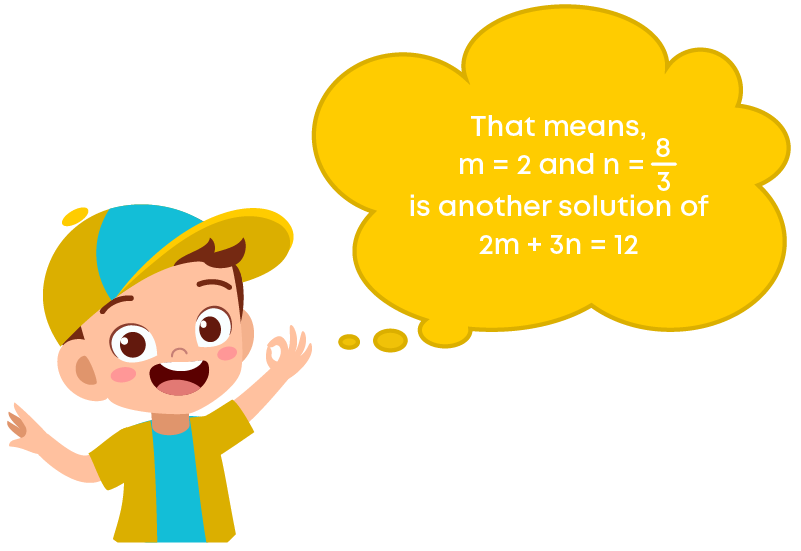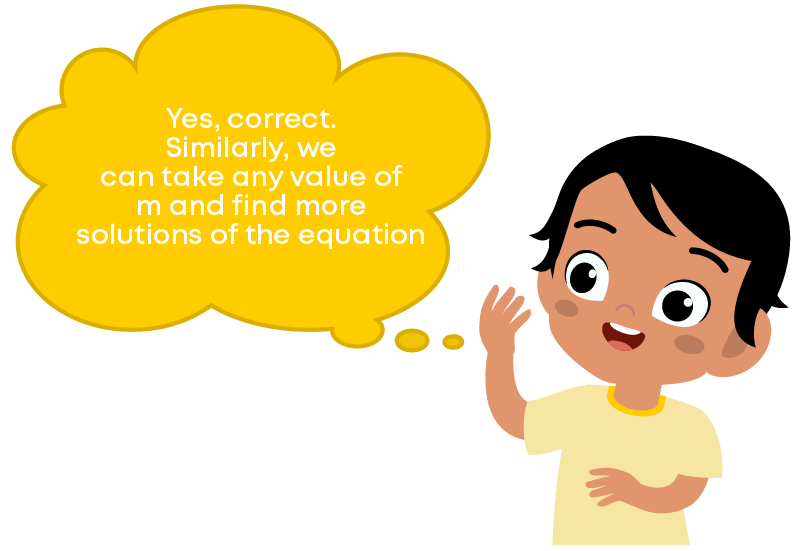What do you understand from the above conversation? We see that there is no end to different solutions of a linear equation in two variables.Let us list out all the solutions that we have found for the equation 2m + 3n = 12.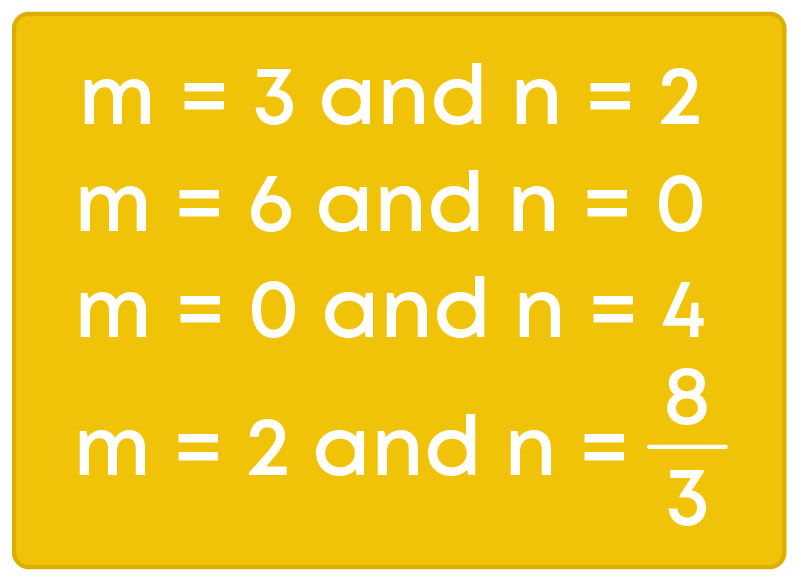These solutions can also be written as an ordered pair.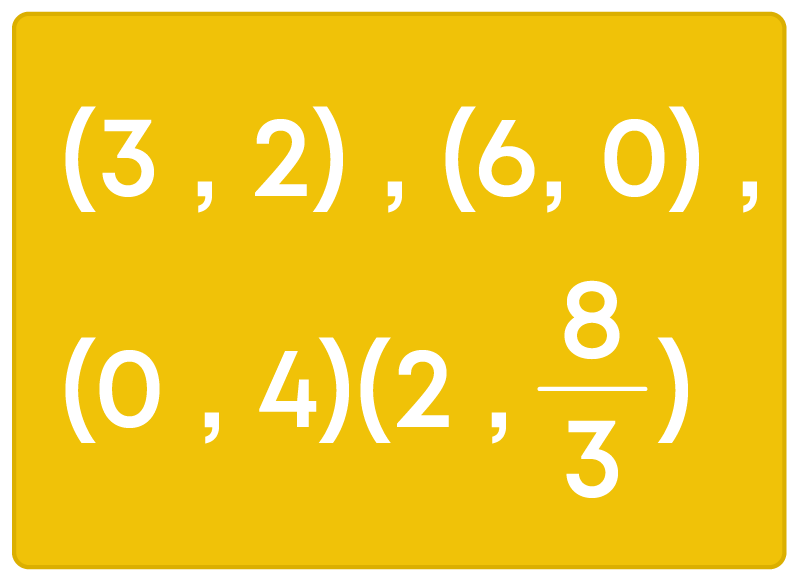That is, first writing the value for ‘m’ and then the value for ‘n’ within simple brackets.

Note: An ordered pair refers to a number written in a certain order. An ordered pair is used to show the position on a graph, where the "x" (horizontal) value is first, and the "y" (vertical) value is second.

Let ax + by = c = 0 be a given linear equation of variables ‘x’ and ‘y’. Then, a pair of values, one for ‘x’ and one for ‘y’, which satisfy the given equation, is called its solution.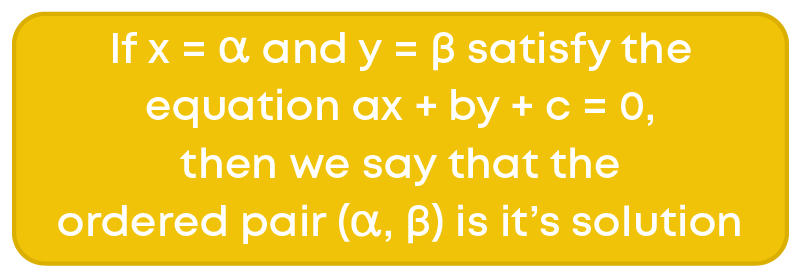To find the solution/s of the given equation we assign some values to one of the variables and then solve the equation to get the second variable. To find the unknown value, we substitute the given solution of the equation and then solve the equation.

#### Graph of a Linear Equation in Two Variables

For a linear equation in two variables, every point on the line will be a solution to the equation and every solution to the equation will be a point on a line. Therefore, every linear equation in two variables can be represented geometrically as a straight line in a coordinate plane. Points on the line are the solutions of the equation. This is called the graph of the linear equation.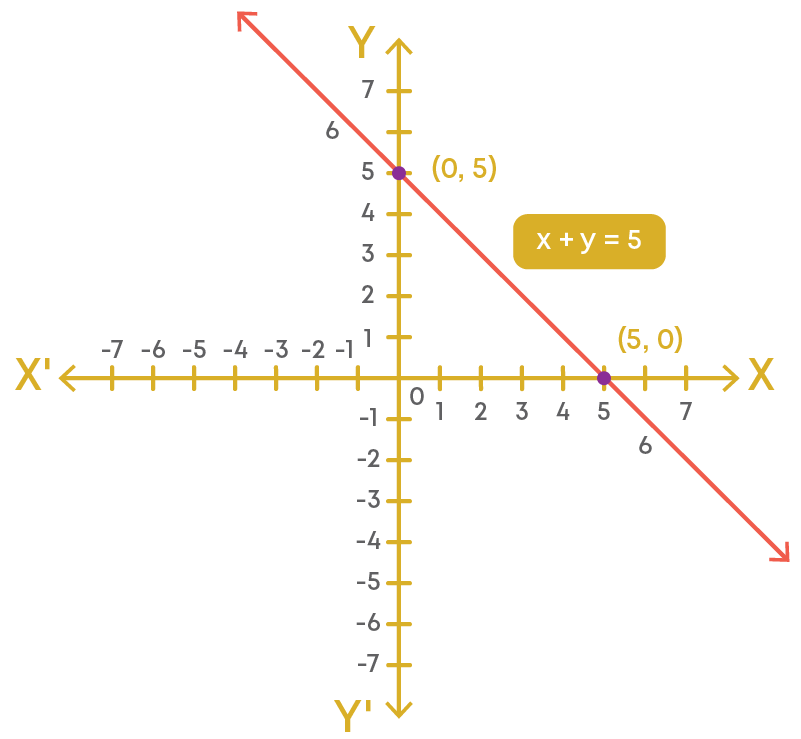We follow the given steps to draw a graph of a linear equation: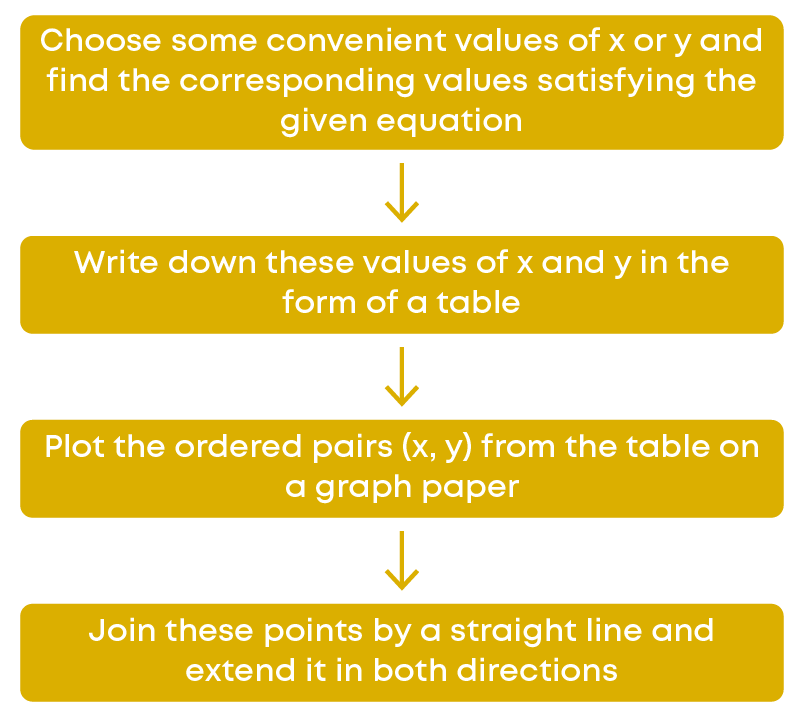We can find the equation of a line passing through a set of points. Let us see how!

Give the equations of two lines passing through (2, 10). How many more such lines exist, and why?

We are given points x = 2 and y = 10. Now let us consider the given equations: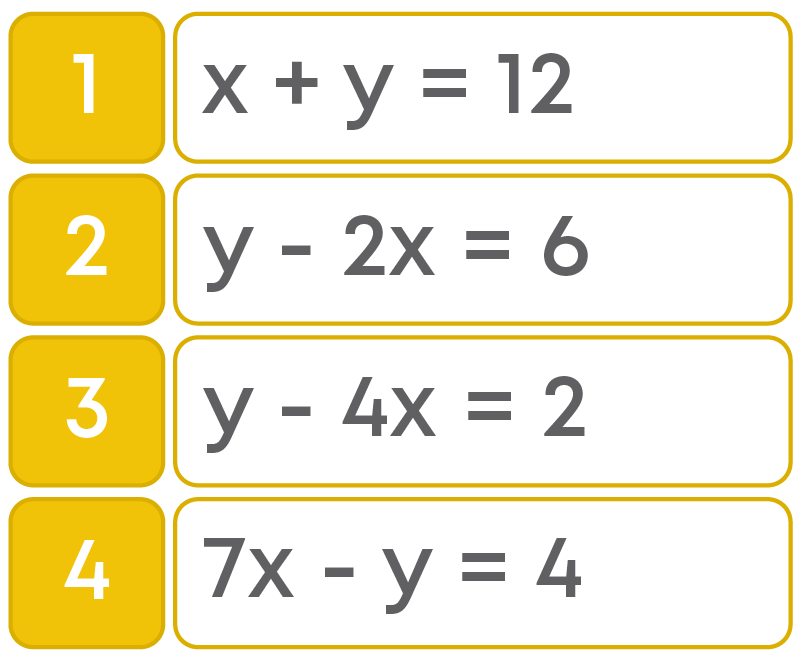How can we check that the given equations have the solution as (2, 10)?

It is simple, we need to substitute x = 2 and y = 10 in the given equations. If these values satisfy the equation, then that point is the solution.

Let us check in equation x + y = 12.

2 + 10 = 12, hence, (2, 10) is its solution.

Similarly, we check in y – 2x = 6.

10 – 4 = 6, hence, (2, 10) is its solution.

So, the equations of two lines passing through (2, 10) are x + y = 12 and y – 2x = 6.

We know that infinite lines can pass through a point. So, there are infinite lines passing through (2, 10).

We can follow the same process for the remaining two equations as well.

Remember: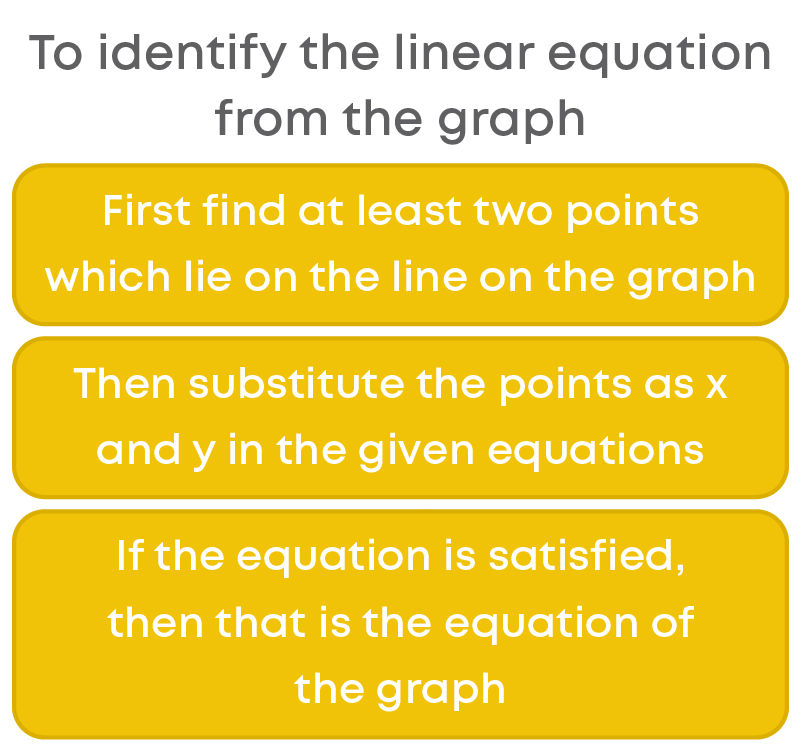Consider the graph given below.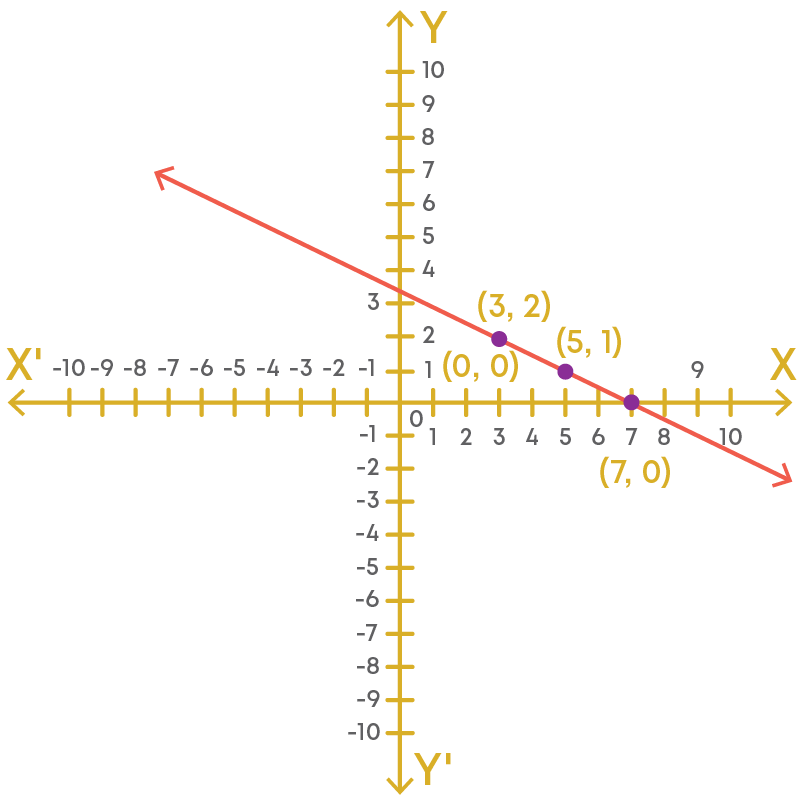Let us find which of these equations given below represent the graph.

• (i) 2y – x = 5
• (ii) x + 2y = 7i

Step 1: Find at least two points which lie on the graph

From the graph, we can see that the given points are (3, 2), (5, 1), and (7, 0).

So, we can consider any two points. Let us consider the points (3, 2) and (7, 0).

Step 2: Substitute the points as x and y in the given equations.

If the equation is satisfied, then that is the required equation of the graph. Now, substitute the values for ‘x’ and ‘y’ from the considered points in the given equations.

For 2y – x = 5,

At (3, 2), x = 3 and y = 2

LHS = 2 × 2 – 3 = 4 – 3 = 1

LHS ≠ RHS

At (7, 0), x = 7 and y = 0

LHS = 2 × 0 – 7 = -7

LHS ≠ RHS

Therefore, 2y – x = 5 is not an equation of the given graph.

For x + 2y = 7,

At (3, 2), x = 3 and y = 2

LHS = 3 + 2 × 2 = 3 + 4 = 7

LHS = RHS

At (7, 0), x = 7 and y = 0

LHS = 7 + 2 × 0 = 7

LHS = RHS

Therefore, x + 2y = 7 is the equation of the given graph.

To draw a graph for a real-life scenario,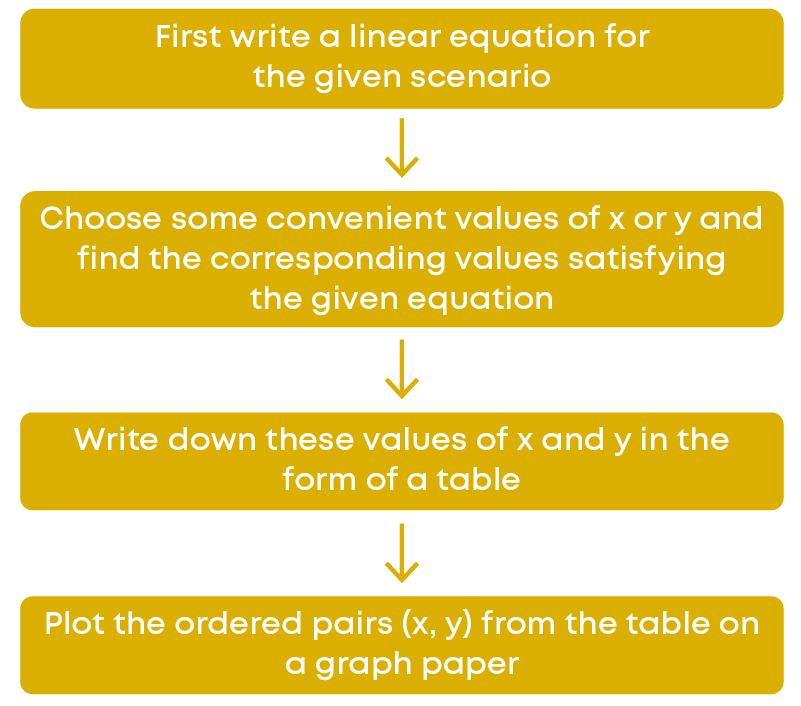Example: Two numbers are such that one of the numbers is double of two more than the other number. Represent this graphically.

Let ‘x’ and ‘y’ be the two numbers. So, we get the equation:

y = 2(x + 2)

Now, let us put x = 0.

y = 2(0 + 2)

y = 4

For y = 0,

0 = 2(x + 2)

x = -2

These values should be plotted on a graph.

Remember, the graph of the equation of the form y = kx is a line which always passes through the origin.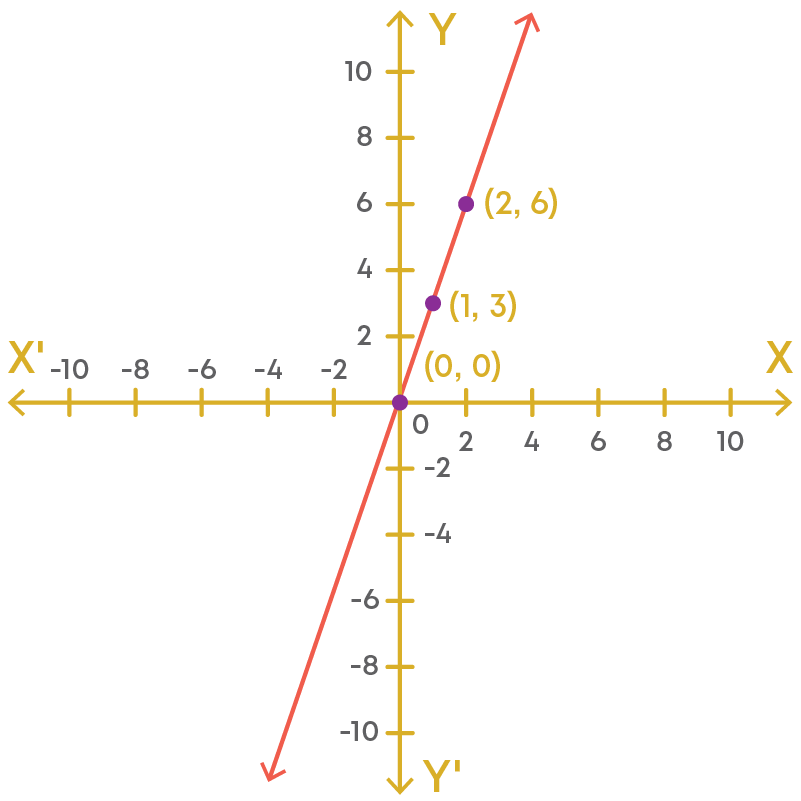Here k = 3.

#### Equations of Lines Parallel To The X-Axis and Y-Axis

Consider the following points and plot them on a graph:

• (2, 0)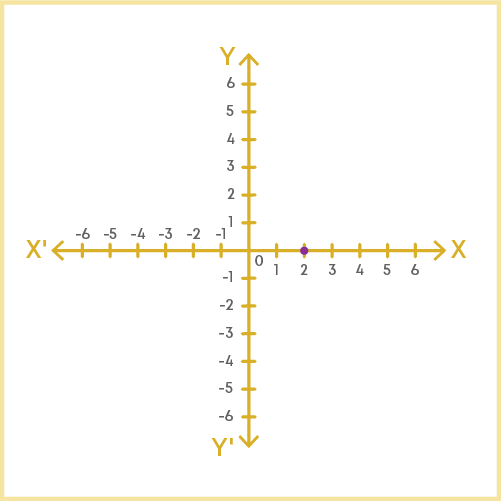• (5, 0)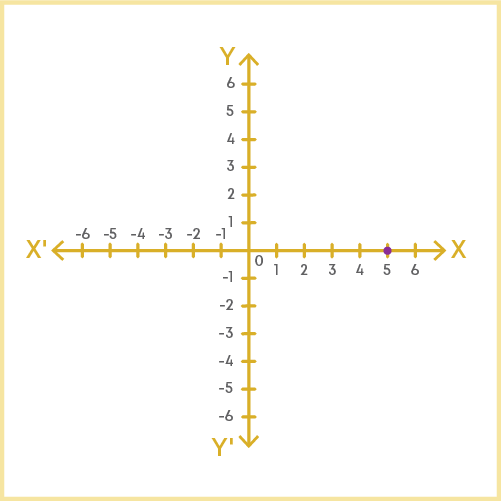• (-4, 0)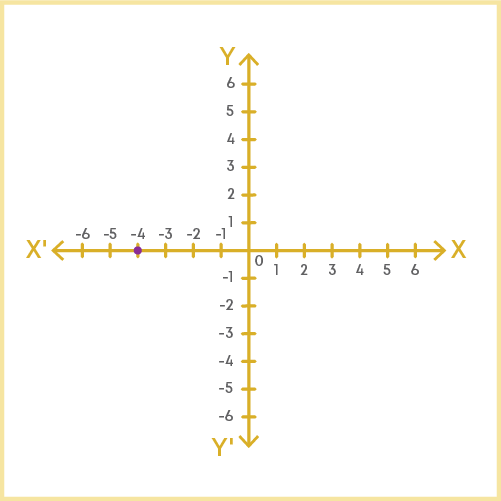All the points lie on the x-axis. But do you know why? For each of the pairs the y coordinate is 0.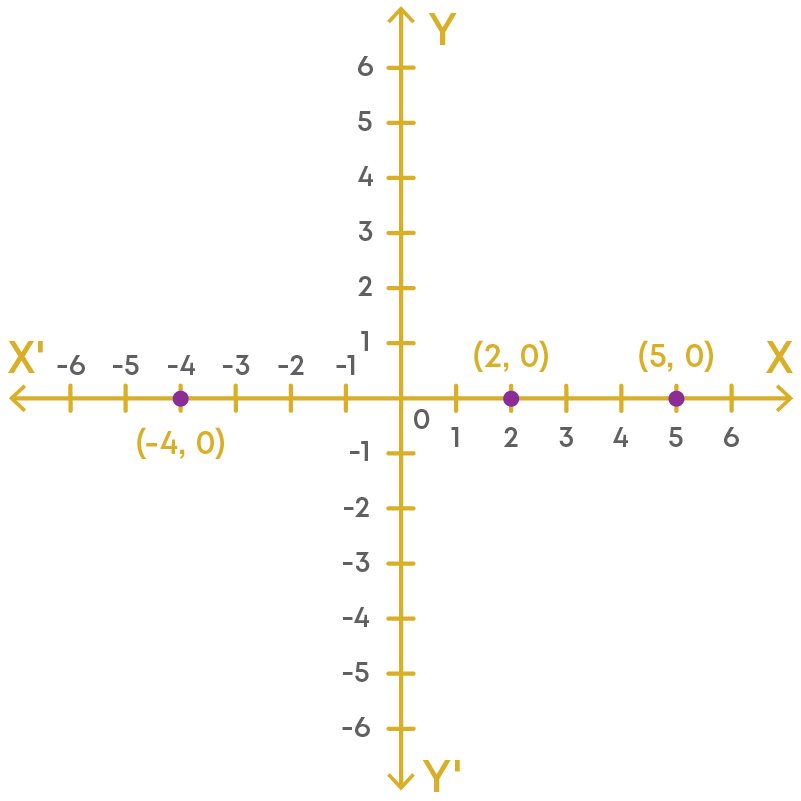Can we say that y coordinate is zero for all the points on the x-axis? Yes! Can you now guess the equation of the x-axis? It is given as: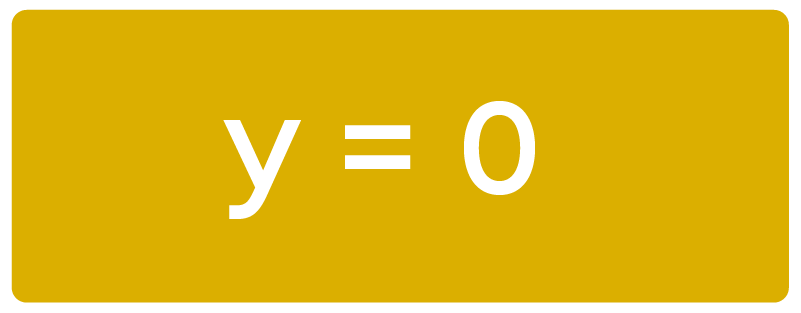Now consider the following points and plot them on a graph:

• (0, 7)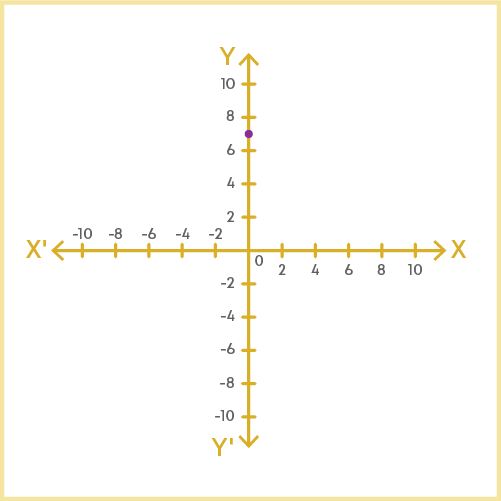• (0, 9)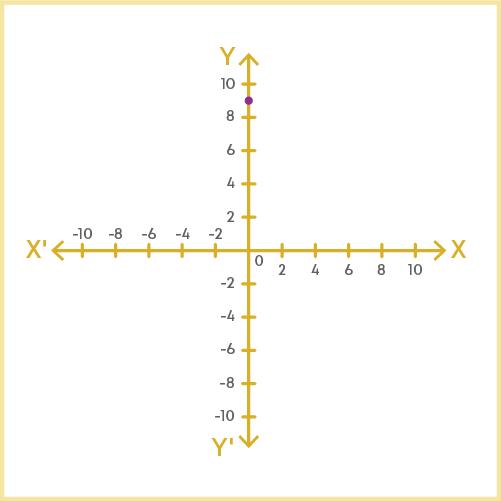• (0, -6)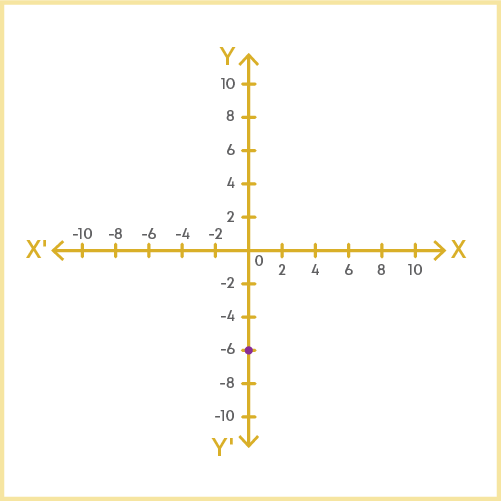All the points lie on the y-axis. But do you know why? For each of the pairs the x coordinate is 0.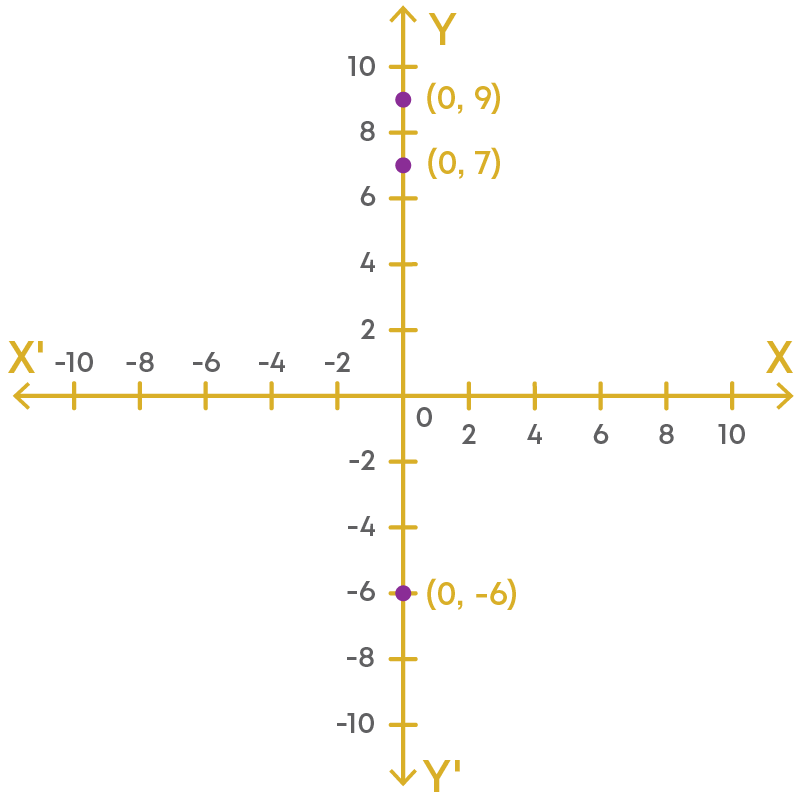Can we say that x is zero for all the points on the y-axis? Yes! Can you now guess the equation of the y-axis? It is given as:Consider the above equation y = 3 which is an equation in one variable; that means it has the unique solution y = 3.

However, when treated as an equation in two variables, it can be expressed as 0(x) + y – 3 = 0.

This has infinitely many solutions. Here, ‘x’ can take any value and ‘y’ is 3. Let us see this geometrically.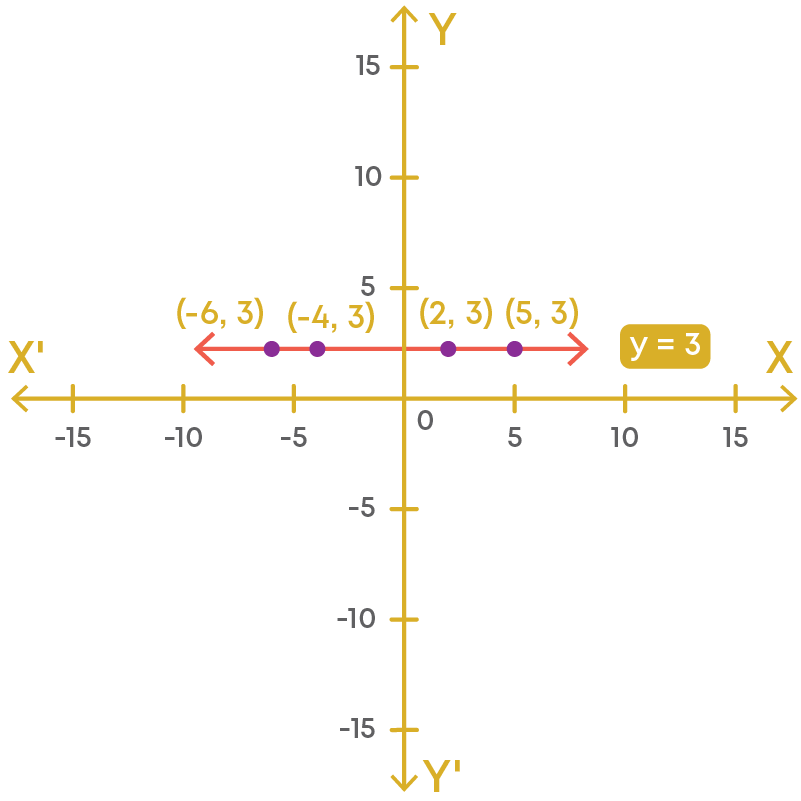Hence, line y = 3 is a line which is parallel to the x-axis. If y is constant and x varies, the line will be parallel to the x-axis.Let us now consider a different equation, say x = 5. Clearly, this is in the form of one variable. How do we convert this equation into an equation with two variables? We take the coefficient of y as 0. So, we can rewrite this as:

x + 0(y) – 5 = 0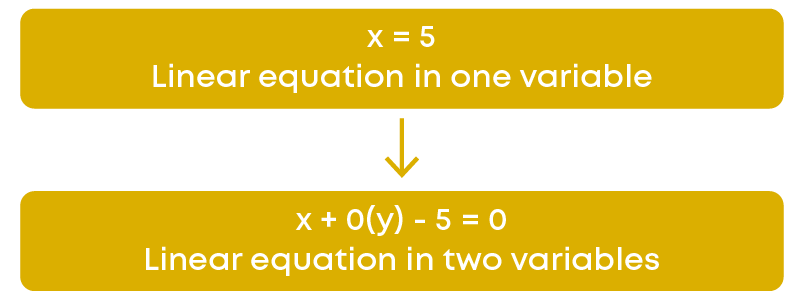Clearly, no matter what value we substitute for y, the value of x will always be 5. Let us see this geometrically.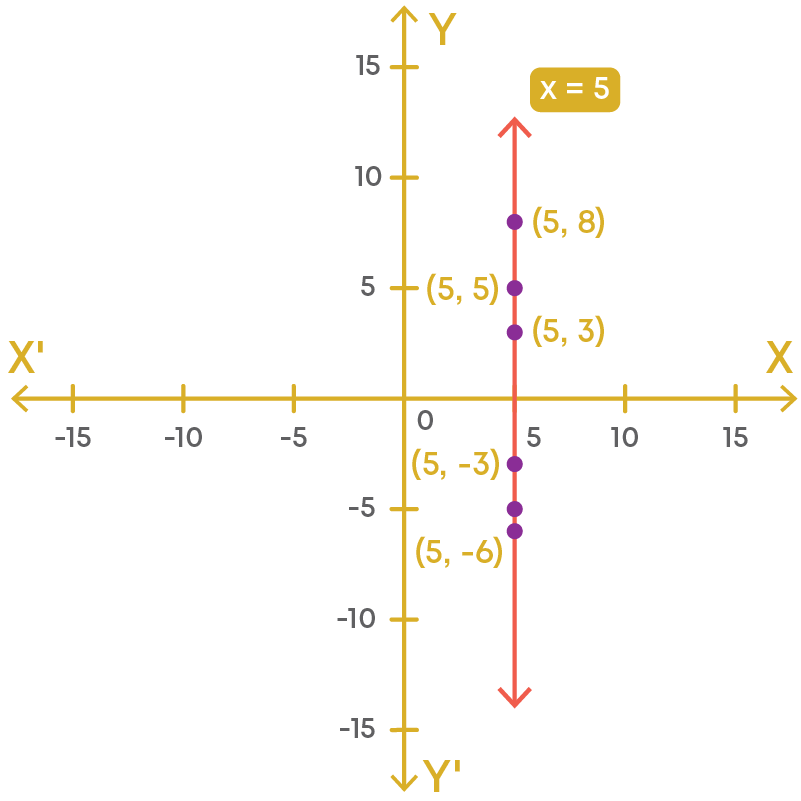Hence, line x = 5 is the equation of a line which is parallel to the y-axis. If ‘x’ is constant and ‘y’ varies, then the line will be parallel to the y-axis.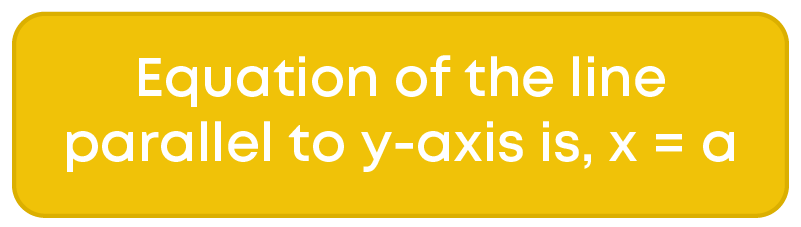To identify the equation of a line parallel to the x-axis, check whether the equation is of the form y = a and whether it cuts the y-axis at the point (0, a), where ‘a’ is a constant.

To identify the equation of a line parallel to the y-axis, check whether the equation is of the form x = b and whether it cuts the x-axis at the point (b, 0).

Can we represent the solution of a linear equation on a number line and the Cartesian plane?

Let us consider the given equation: 2x + 4 = 10

Let us first solve for x.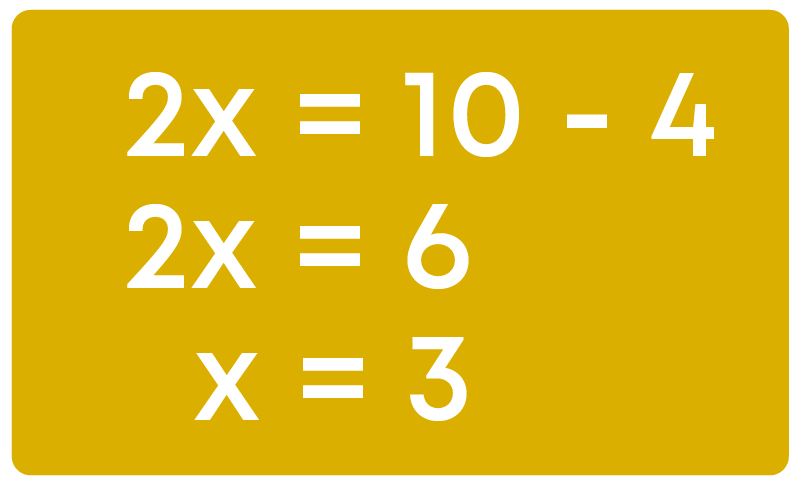Now, let us represent x = 3 on a number line as shown below: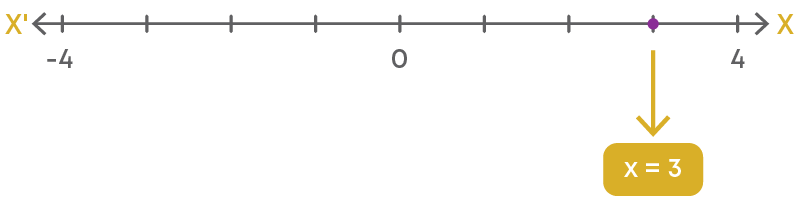Here, the point x = 3 represents the solution of the equation 2x + 4 = 10.

Now how do we plot x = 3 on a cartesian plane?

In a cartesian plane, we have x and y axis. Hence, we write the equation x = 3 in terms of ‘x’ and ‘y’.

x + y = 3

x + 0y = 3

Now, let us find the solution of the equation x + 0y = 3

Here, we can take any value for ‘y' and the value of ‘x’ will remain constant as 3. So, we can take the points as (3, 0), (3, 2), and so on. Now let us see the representation on the cartesian plane: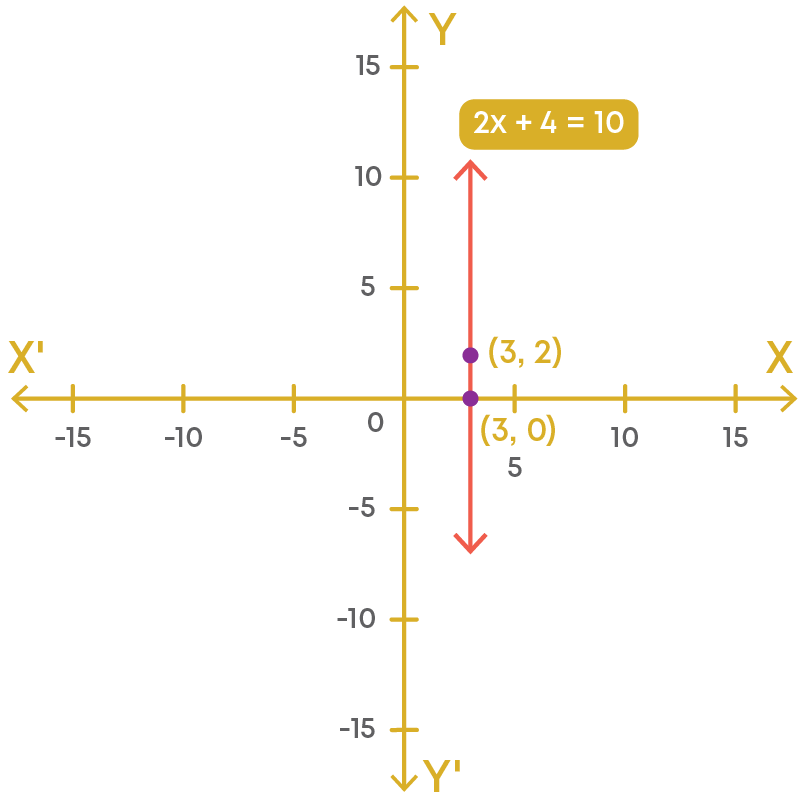Hence, we can see that in two variables, 2x + 4 = 10 is a line parallel to the y-axis.

By now we have understood how to represent an equation on a number line and on a cartesian plane. What do you understand by the geometric representation of an equation as equation in one variable/two variables? It is simple.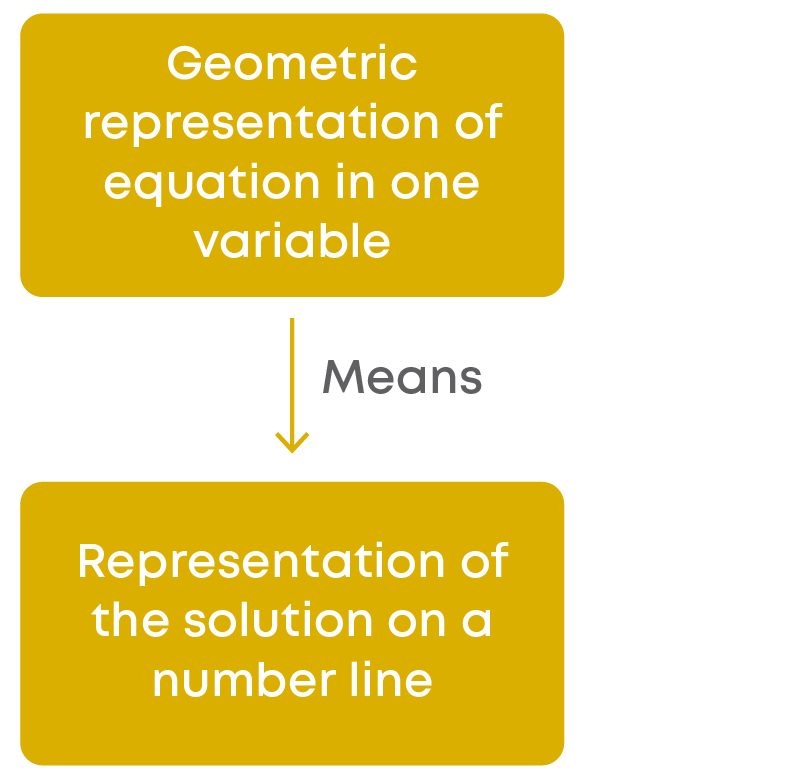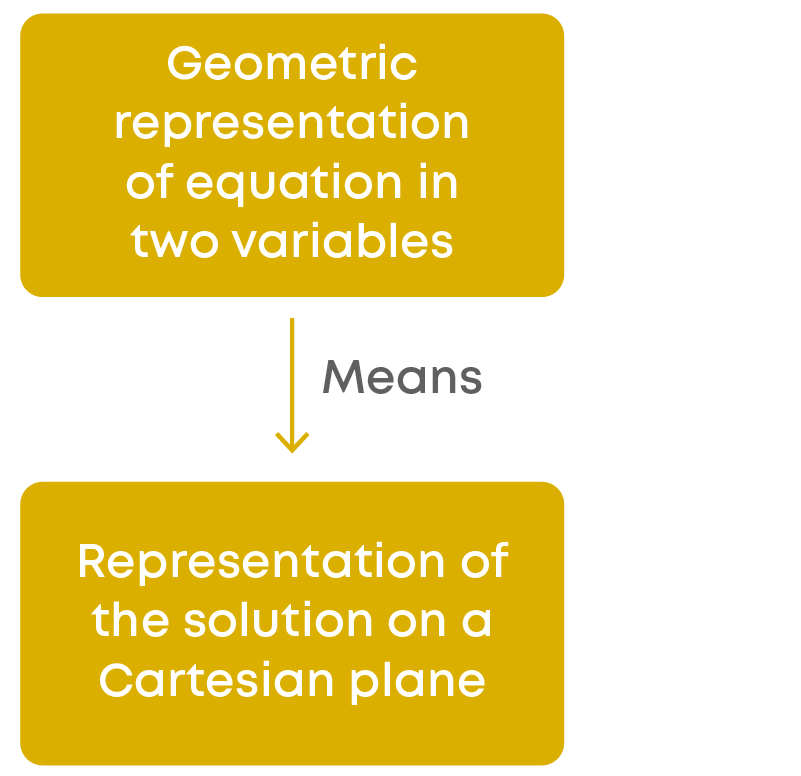To represent the solution on a number line, follow these steps:

• Solve the equation for ‘x’.
• Represent this value of ‘x’ on a number line.

To represent the solution on a cartesian plane, follow these steps:

• Write the given equation as an equation in two variables.
• Since ‘x’ is constant you can take any value of ‘y’ and plot the pairs on a Cartesian plane.
• After plotting the points, join all the points with a line and you will notice that the line is parallel to the y-axis.

#### Common Errors

The following are topics in which students make common mistakes when dealing with linear equations in two variables:

• 1. Identify linear equations in two variables
• 2. Is 5x = 0 a linear equation in two variables?
• 3. Solving linear equation in two variables

#### Identify Linear Equations In Two Variables

An equation of the form ax + by + c = 0, where 'a', 'b' and 'c' are real numbers, such that ‘a’ and ‘b’ are not both equal to zero, is called a linear equation in two variables. The following are not linear equations in two variables.

• 2p2 + 2q = 50: This equation is not in linear form.
• t + u + v = 10: This equation has more than two variables.

The following are linear equations in two variables.

• 2x + 3y = 10
• k + 2q = 50
• 4t + 4t + v = 100

#### Is 5x = 0 A Linear Equation In Two Variables?

We know that an equation of the form ax + by + c = 0, where 'a', 'b' and 'c' are real numbers, such that ‘a’ and ‘b’ are not both equal to zero, is called a linear equation in two variables.

If ‘a’ and ‘b’ both equal to zero, then the equation is not a linear equation in two variables. Hence, 5x = 0 is a linear equation in two variables.

5x = 0 can be written as 5x + 0y = 0.

#### Solving Linear Equation In Two Variables

To find the solution/s of the given equation we assign some values to one of the variables and then solve the equation to get the second variable. For example:

The following is the correct method

4x + 2y = 6

when x = 0

0 + 2y = 6

y = 3

(0, 3) is one of the solutions of the given equation.

The following is not the correct method

4x + 2y = 6

4x = 6 - 2y

x = (6 - 2y) ÷ 4

((6 - 2y) ÷ 4, 0) is one of the solutions of the given equation.

#### Conclusion

Can you solve this riddle after learning all about linear equations?

Considering the value of ‘x’ as consecutive whole numbers, give four solutions for the equation y = 4x.#### Arpana

Author

Arpana is an education specialist with years of teaching Math and developing content. She previously worked as a freelance content developer, developing lesson plans for a reputed publisher of text books and content for various educational companies. She is an enthusiastic teacher who has taught students from India, the UK, and New Zealand.